# NCERT Solutions for Class 8 Maths Chapter 12 Exponents and Powers Ex 12.2

NCERT Solutions for Class 8 Maths Chapter 12 Exponents and Powers Exercise 12.2

Ex 12.2 Class 8 Maths Question 1.
Express the following numbers in standard form:
(i) 0.0000000000085
(ii) 0.00000000000942
(iii) 6020000000000000
(iv) 0.00000000837
(v) 31860000000
Solution: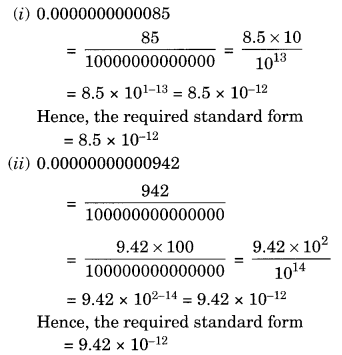(v) 31860000000
= 3.186 × 10000000000
= 3.186 × 1010
Hence, the required standard from = 3.186 × 1010

Ex 12.2 Class 8 Maths Question 2.
Express the following numbers in usual form.
(i) 3.02 × 10-6
(ii) 4.5 × 104
(iii) 3 × 10-8
(iv) 1.0001 × 109
(v) 5.8 × 1012
(vi) 3.61492 × 106
Solution: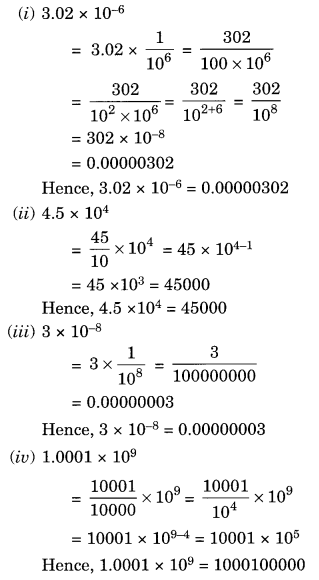Ex 12.2 Class 8 Maths Question 3.
Express the number appearing in the following statements in standard form.
(i) 1 micron is equal to $$\frac { 1 }{ 1000000 }$$ m.
(ii) Charge of an electron is 0.000,000,000,000,000,000,16 coulomb
(iii) Size of a bacteria is 0.0000005 m
(iv) Size of a plant cell is 0.00001275 m
(v) Thickness of a thick paper is 0.07 mm.
Solution: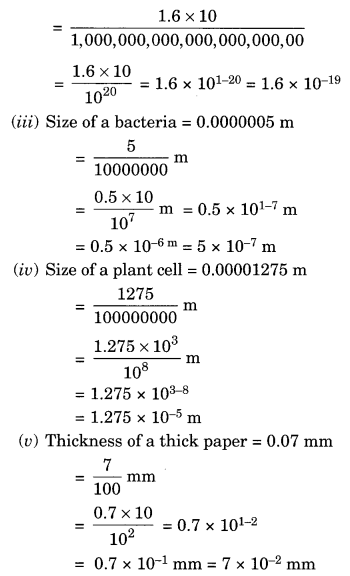Ex 12.2 Class 8 Maths Question 4.
In a stack there are 5 books each of thickness 20 mm and 5 paper sheets each of thickness 0.016 mm. What is the total thickness of the stack?
Solution:
Thickness of books = 5 × 20 = 100 mm
Thickness of 5 paper sheets = 5 × 0.016 mm = 0.080 mm.
Total thickness of the stack = 100 mm + 0.080 mm = 100.080 mm = 1.0008 × 102 mm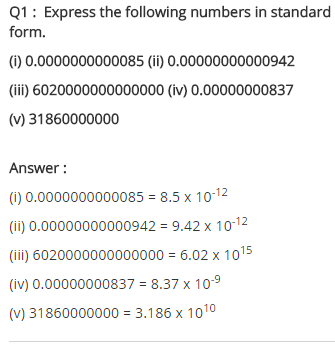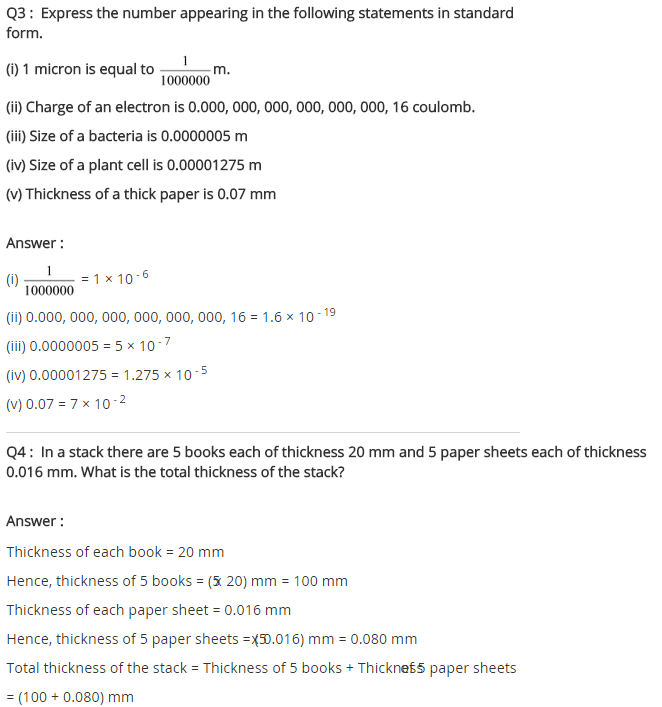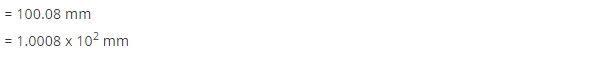+